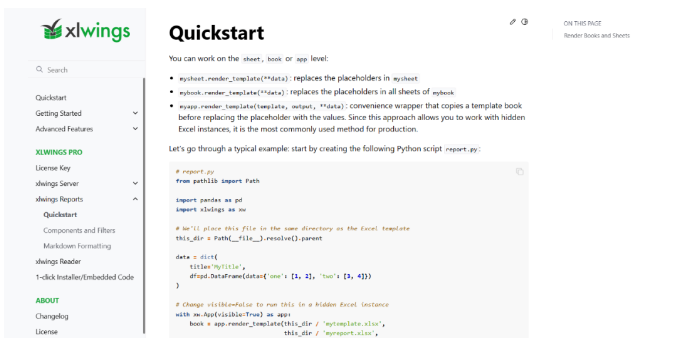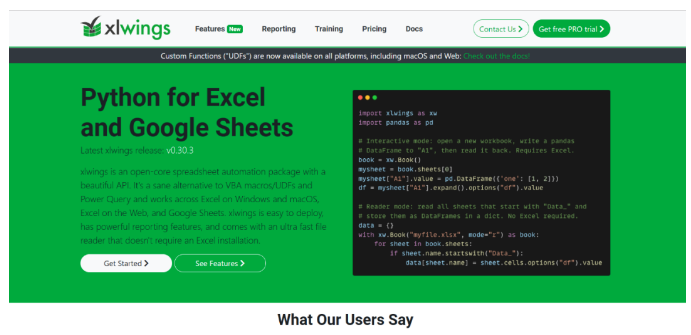2023
09-08

# python数据分析三剑客遇上xlwings教程Excel作为我们日常办公过程中必备的数据处理软件，几乎天天在用。很多python模块也对Excel提供了API支持，比如之前说过的pandas、openpyxl等都对Excel的操作提供了很好的支持。

https://docs.xlwings.org/en/latest/xlwings作为python的非标准模块，需要使用pip的方式安装一下该模块。

pip install xlwings

1、xlwings处理Excel数据

# 导入xlwings模块
import xlwings as xlw

# 读取Excel文档数据对象
work_book = xlw.Book('/usr/local/test.xlsx')

# 获取Excel的工作表sheet对象
sheet_ = work_book.sheets["sheet1"]

# 打印工作表的绝对路径地址
print(work_book.fullname)

# 打印工作簿的名称
print(sheet_.name)

# 读取当前工作表的某个单元格的内容
print(sheet_.range('A1').value)

# 写入当前工作表的某个单元格的内容
sheet_.range('A1').value = 'Python 集中营'

# 清除某个单元格的内容和格式
sheet_.range('A2').clear()

# 在某个单元格中输入Excel的计算公式
sheet_.range('A3').fornula = '=SUM(B6:B7)'

# 获取单元格中的公式
print(sheet_.range('A4').formula_array)
2、xlwings配合三剑客使用

xlwings模块支持通过numpy模块的array函数可以直接将数组的序列化数据直接写入到某个单元格中。

# 导入numpy模块，用于创建array数组
import numpy as np

# 初始化numpy模块的数组对象
array_data = np.array(('a', 'b', 'c'))

# 将array数组对象直接赋值给某个单元格
sheet_.range('A5').value = array_data

# xlwings模块支持将pandas.DataFrame数据对象直接写入到
# 某个单元格中。

# 导入pandas模块，用于处理DataFrame对象的数据
import pandas as pd

# 初始化pandas.DataFrame数据对象
data_frame = pd.DataFrame([['a', 'b'], ['c', 'd']], columns=['name', 'value'])

# pandas.DataFrame数据对象赋值给某个单元格
sheet_.range('A6').value = data_frame

# xlwings模块读取并返回DataFrame对象
data_frame2 = sheet_.range('A7').options(pd.DataFrame, expand='table').value

# 通过matplotib模块创建图表对象绘制，然后使用xlwings模块将图表对对象保存
# 到Excel的文件对象中。

# 导入matplotlib图表绘制模块
import matplotlib.pyplot as plt

# 创建Figure图表对象
fig_ = plt.figure()

# 绘制图表
plt.plot(['a', 'b', 'c', 'd'])

# 将Figure图表对象写入到Excel对象中

https://www.xlwings.org/3、2023-04-15粉丝分享排行榜